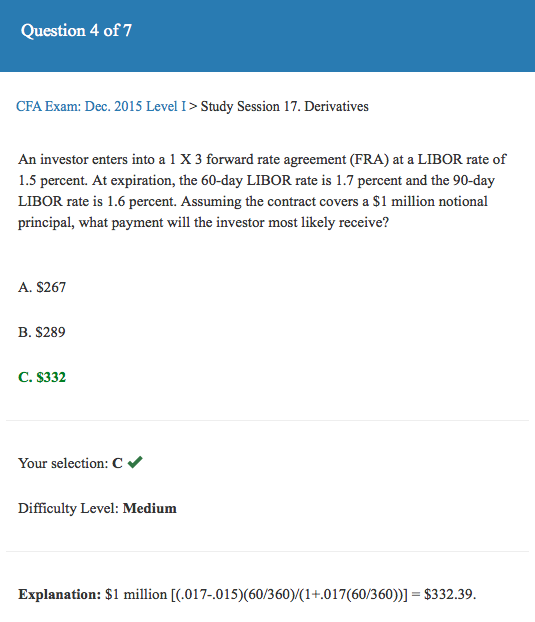CFA CFA Level 1 Calculation of FRA

# Calculation of FRA

• Author
Posts
• 1

Does anyone know how the fra formula work? I keep getting \$1967 as an answer. This is a sample question from Analyst Notes for level 1 CFA. Thank you in advance 🙂• 4

So, a 1 x 3 Fra means the contract will expire 30days and will be based on the 60day LIBOR 30 days from now.

where you may be going wrong is the interest rate is annualised, so you will need de-annualise to the 60/360 rate. I believe you are taking the .2% * 1 million notional value; however, see below.

you agreed to enter into a contract at a rate of 1.5% and the market rate 30 days later is 1.7%. So it is good for you.

The difference between the two rates is .2%. However, this is an annualised rate. .002 * 60 / 360 = .000333

1 million * .000333= \$333.33

however we are going to settle at the contract expiration so we need to discount the 333.33 (which is 60 day future value) back to get the present value by today’s market rate of 1.7% (remember to de annualise):

333.33/1 + .017 * 60/360 = \$332.3883438

Hope this helps,

-Ron